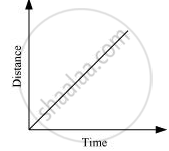# Show the Shape of the Distance-time Graph for the Motion in the Following Cases: a Car Moving with a Constant Speed. - Science

Show the shape of the distance-time graph for the motion in the following cases:

A car moving with a constant speed.

#### Solution

A car moving with a constant speed covers equal distance in equal intervals of time.

Such motion of car is represented in the given distance-time graph.Is there an error in this question or solution?

#### APPEARS IN

NCERT Class 7 Science
Chapter 13 Motion and Time
Q 7.1 | Page 156